This is the third post in the series of posts titled “The Anatomy of a JavaScript function”.Photo by Nick Fewings on Unsplash.com

If you are brand new to functions in JavaScript, I warmly recommend reading part 1 and part 2 first.

Here’s an overview of what we learned in the first two parts:

Javascript functions are like machines that we can build and run. We can define their inputs and outputs.

In the first article in this series, we built an example “machine”. In the second article, we built another, similar one - and then we saw how to generalize them.

So far, for all our examples, we were using ES5 syntax - the “traditional” JavaScript.

Now that we’ve got the very basics of functions down, we can have a look at differences between the ES5 and ES6 function syntax.

## Comparing ES5 and ES6 functions in JavaScript

The differences in syntax between ES5 and ES6 functions are based on two main factors:

1. Number of parameters that the function accepts: multiple params, a single param, or no params?
2. Is the function body spanning a single line or multiple lines?

If we combine the three possibilities in the number of params, with the two possibilities in the length of the function body, we get 6 different situations:

1. Multiple parameters, multi-line function body
2. Multiple parameters, single-line function body
3. Single parameter, multi-line function body
4. Single parameter, single-line function body
5. No parameters, multi-line function body
6. No parameters, single-line function body

Next, we’ll see an example of each one.

### Function definitions with multiple parameters

Let’s begin by looking at a simple function in ES5:

``````var a = function(num1, num2) {
var subtract = num1 - num2;
return subtract > 0;
}
``````

In the above code, there are two important things to note:

1. The function definition has more than one parameters (`num1` and `num2`); we can say it has multiple parameters
2. The function body - the code between the opening curly bracket, `{`, and the closing curly bracket `}` - spans on more than one line; it spans on multiple lines

So, the above function definition has multiple parameters and it’s body spans multiple lines.

A function such as the one above would be almost identical in ES6, syntax-wise: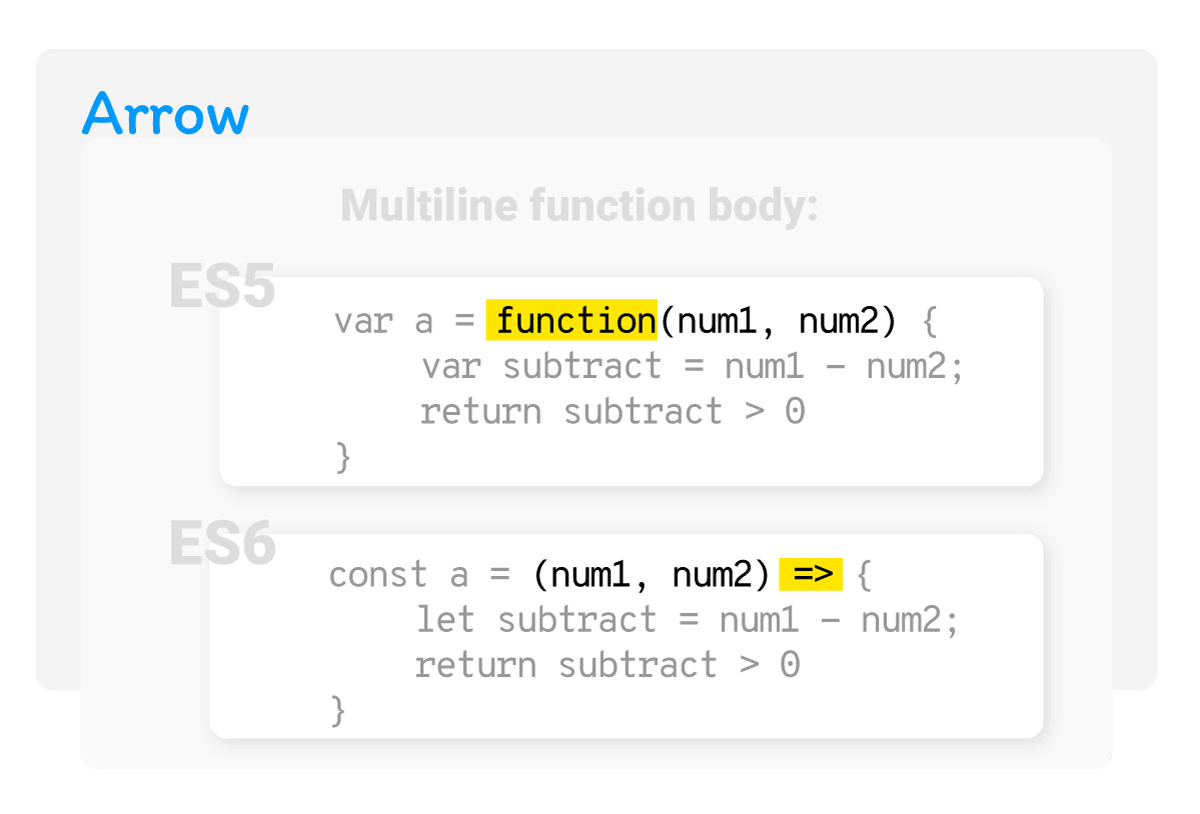.

Looking at the above image, we can see that the only difference between the two is that the word `function` in ES5 was replaced with the fat arrow, `=>` in ES6.

Let’s now look at the exact same function, only this time we’ll shorten the function body to a one-liner:

``````var a = function(num1, num2) {
return (num1 - num2) > 0
}
``````

This time, in ES6, besides replacing the `function` keyword with a `=>`, we’ll also remove the curly brackets: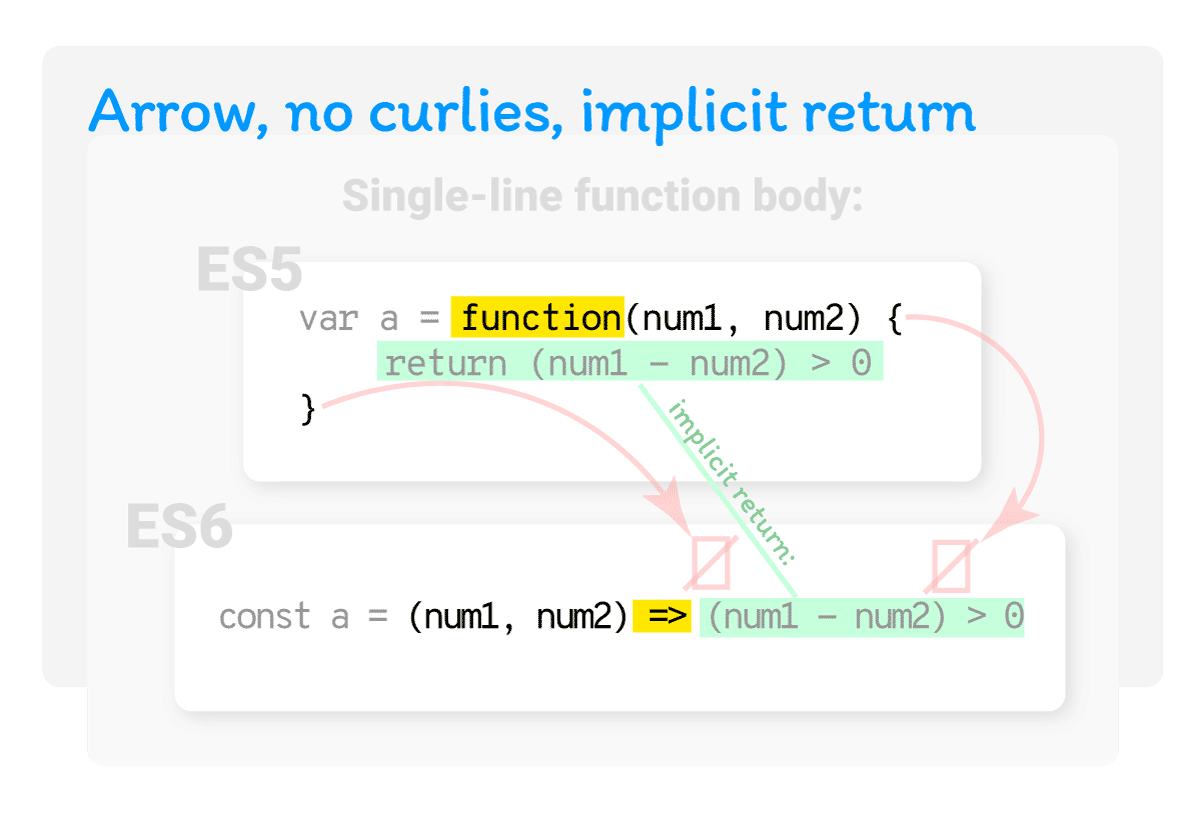.

Also, notice the implicit return: in the ES6 version of this function, we don’t have to write the `return` keyword.

This effectively turns a three-line ES5 function into a one-line ES6 function.

We’ve just covered two cases with multi-line parameters in function definitions, and we’ve got four more to go.

## Function definitions with a single parameter

Let’s now define a function with one parameter, `num`:

``````var a = function(num) {
var b = num > 0;
return b
}
``````

If we are to convert it to ES6, this is what it would look like: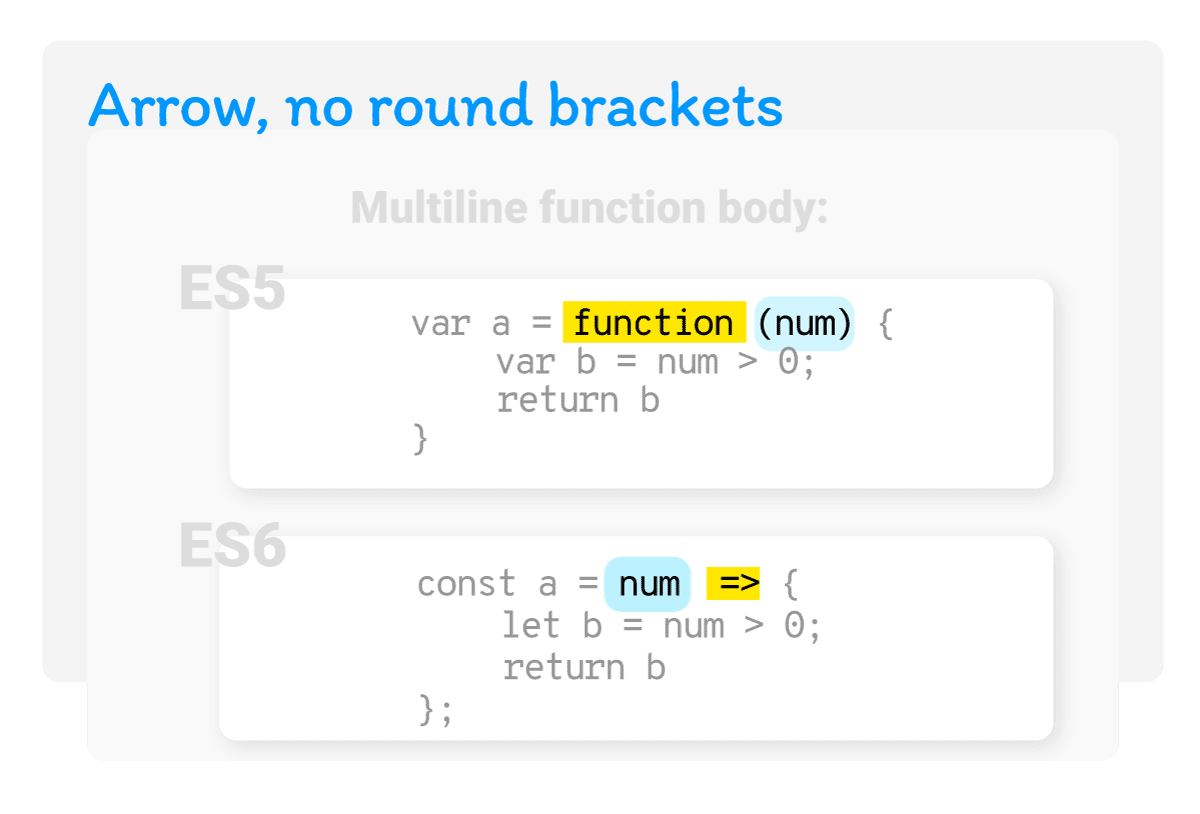.

Contrary to a function definition with multiple parameters, in an ES6 function that has only a single parameter, we don’t use the round brackets around the parameter.

If we take the above ES5 function and simplify the function body so that it becomes a one-liner, it would look like this in ES5:

``````var a = function(num) {
return num > 0
}
``````

In ES6, our single parameter, single-line function body would update to this: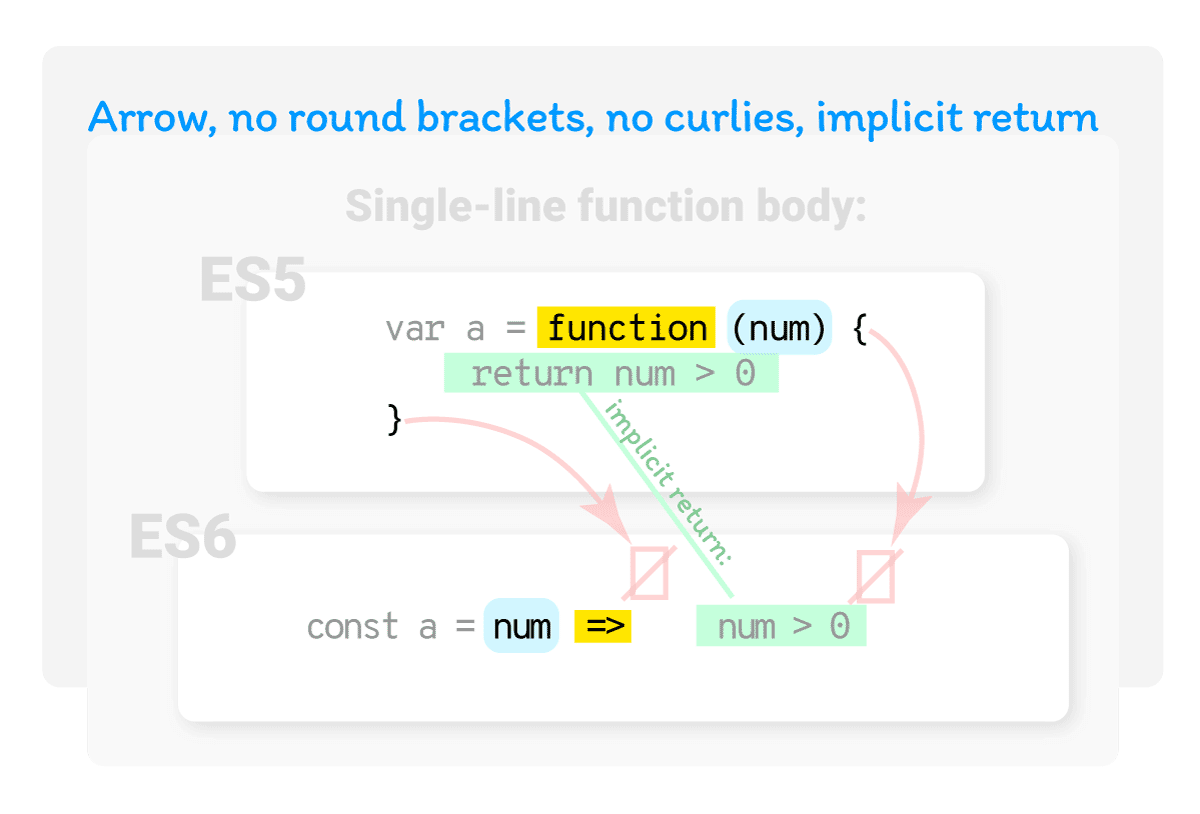.

Again, a three-line function definition fits onto a single line.

All that’s left to cover now is the difference between the ES5 and ES6 function syntax when the function definition has no parameters.

## Function definitions with no parameters

We have two cases here: a multi-line function body, and a single-line function body.

Here’s an ES5 function with a multi-line function body with no parameters:

``````var a = function() {
var b = num > 0;
return b;
}
``````

This is what the update to ES6 looks like: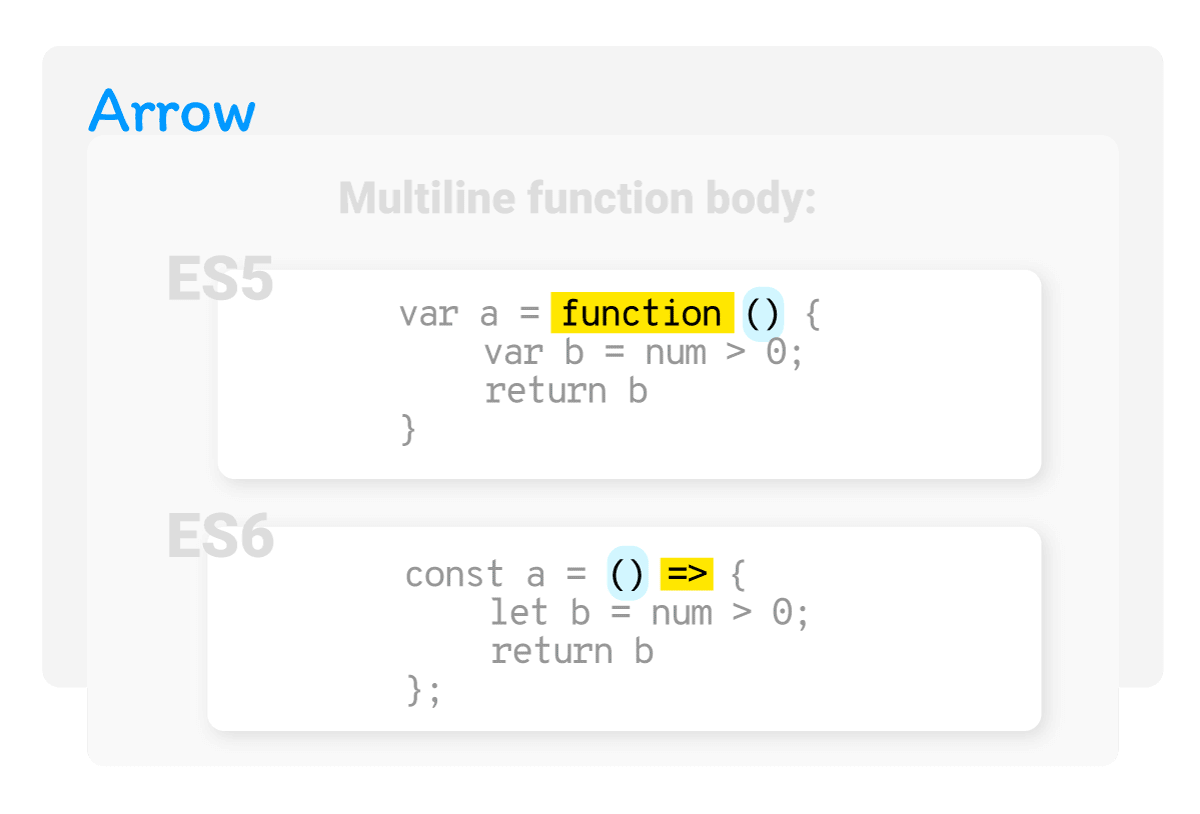.

Here’s an ES5 function with a single-line function body and no parameters:

``````var a = function() {
return num > 0
}
``````

In this case, we get a really minimalistic function definition in ES6: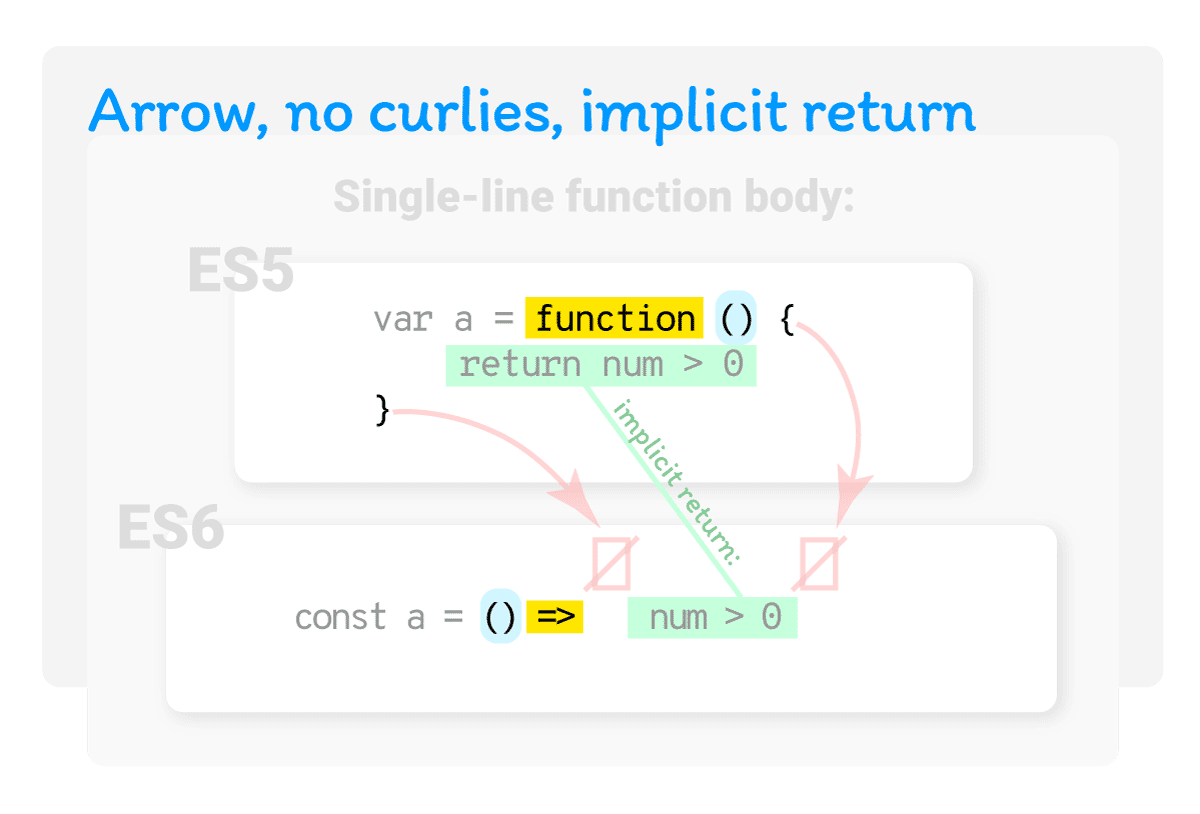.

Finally, here’s an overview of ES5 to ES6 conversion with all the possible combinations in a single image.

Lets look at the above image here as well: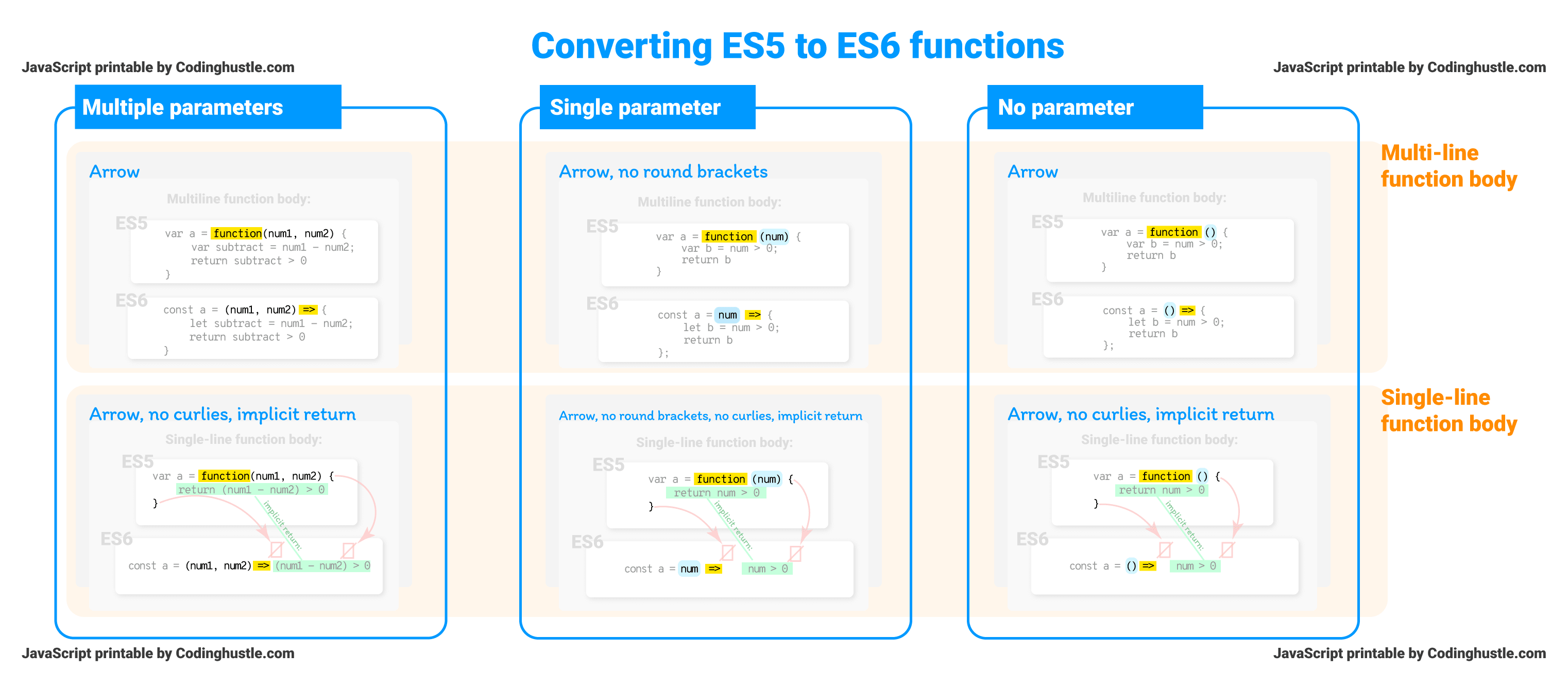.

As we can see above, we could group our functions by number of parameters:

• multiple parameters
• single parameters
• no parameter

We could also group them by whether it’s a multi-line function body, or a single-line function body.

Viewing things like this leads us to the following conclusions.

If the function body is multi-line:

The `function` keyword BEFORE the parameters,
becomes the fat arrow AFTER the parameters.

If the function body is single-line:

The ES5 curlies are always omitted in ES6, and
The ES5 `return` keyword is always implicit in ES6

Finally, regardless of the length of the function body, if an ES5 function has a single parameter:

The brackets around an ES5 function’s single parameter will be removed when written as an ES6 function.

## Converting ES5 to ES6 functions (and vice-versa)

To convert ES5 to ES6 functions, we use the tool known as Babel.

Just type in your ES5 function, and you’ll get the output written in the ES6 format.

To do the opposite - that is, to convert an ES6 function into an ES5 function, you can use the tool such as Lebab.

That’s it for part 3 in this series of articles titled: The Anatomy of a JavaScript function.

In part 4, we’ll look at function arguments in detail.